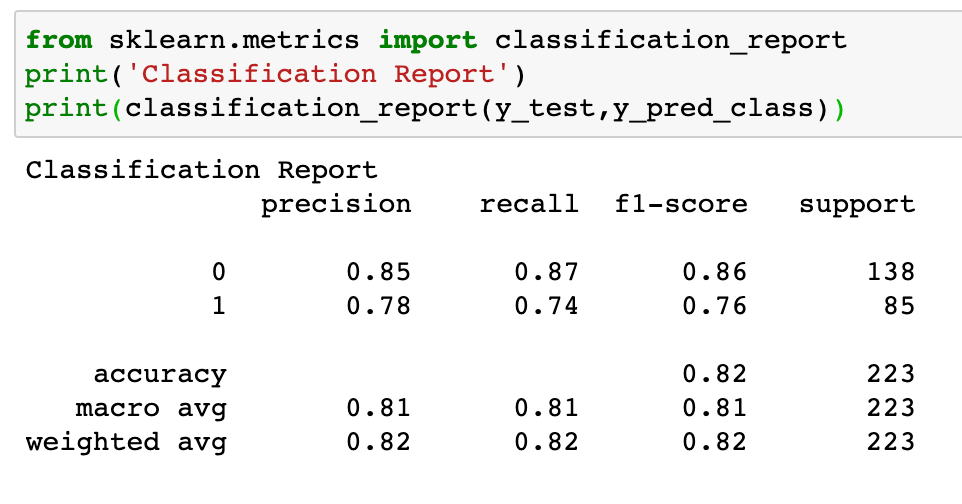# Evaluation Metrics

`; print((TP + TN) / (TP + TN + FP + FN))orprint(metrics.accuracy_score(y_test, y_pred_class))Cat Example Answer0.82062780269058382% Accuracy`
`print(TP / (TP + FP))orprint(metrics.precision_score(y_test, y_pred_class))Cat Example Answer0.7777777777777778`
`print(TP / (TP + FN))orprint(metrics.recall_score(y_test, y_pred_class))Cat Example Answer0.7411764705882353`
`print(TN / (TN + FP))Cat Example Answer0.8695652173913043`
`print(FP / (TN + FP))Cat Example Answer0.13043478260869565`Above is some helpful code utilizing scikit-learn to help get a good understand of your Classification Model. Note: We are trying to (1, or Cats) in the above example

--

--